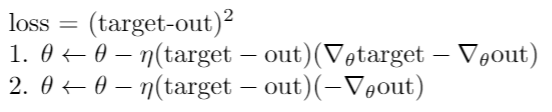Hi, I wanted to ask if PyTorch considers gradient w.r.t target in it’s computation. Consider the following example:
Here the target as well as output both comes from the same network

``````net = torch.nn.Linear(2,2)
input = torch.tensor([1.,0.])
out = net(input)
target = net(torch.tensor([2.,2.]))
loss = nn.functional.mse_loss(out,target)
loss.backward()
optimizer.step()
``````Does the above code translates to the gradient update of 1 or 2?
If it translates to 2 then how should I implement so that the gradient update is as given in 1?
Basically I want to update network parameters considering the gradient wrt to both out as well as target.

Thanks!
Any help would be really appreciated.

Hi,
yes, PyTorch considers gradients w.r.t. the output as well as the target in your case.

Any quick way to check this?

Using backward hooks.Hey hi, thanks a lot! Used hooks and did some maths and yeah you’re correct. Idk why at first instance I thought that `mse_loss` treats/expects targets as constant.

1 Like

Hi,
glad to hear you’ve been able to confirm my answer! Don’t feel bad, you’ve learned something!